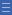## Featured resource

Home > Topdrawer > Fractions > Misunderstandings > Fractions as a double count

# Fractions as a double count

Fractions as a double count

It is common for students to see the numerator and the denominator as two separate whole numbers, recording a count of two different things.

Tasks such as these are usual in classrooms.

 Colour two thirds of this shape. What fraction of this shape is shaded?

Students learn to connect a part-whole area diagram with the written fraction by thinking, "The denominator tells how many parts, and the numerator tells how many are shaded."

While this is a useful basic strategy, it is problematic for several reasons.

• The written fraction is seen as a pair of whole numbers, each recording the count of something different: a 'double count'.
• Many students believe this double count to be the actual definition of a fraction.
• A fraction is not comprehended as a single number, having a value like other numbers.
• The double count cannot be generalised to all situations, such as "Share two pancakes equally among four people."
• Students can lose sight of the whole and interpret a part-whole situation as a ratio, especially when working with fractions of collections.

Yes

Yes

Name Class SectionWrong number Folder 17Expanding the view Folder 17Fraction fiddle Folder 17Year 3: Model and represent unit fractions including 1/2, 1/4, 1/3, 1/5 and their multiples to a complete whole Infobox 3Year 2: Recognise and interpret common uses of halves, quarters and eighths of shapes and collections Infobox 3Year 4: Investigate equivalent fractions used in contexts Infobox 3Source Infobox 3